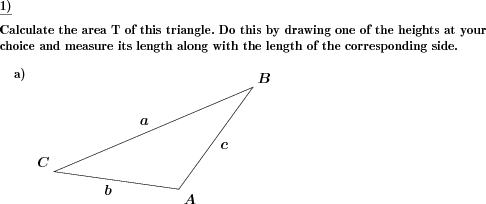Custom math worksheets at your fingertips# Details for problem "Area of triangle draw height and measure"

Quickname: 2915

Junior High School, Middle School, High School.

## Summary

A triangle is given. Draw a height of choice, measure side and height and calculate area.

## Example## Description

A triangle is shown. The task is to

1. draw any height
2. measure the length of the height ha and the corresponding side a
3. use the formula T = a * ha / 2 to calculate the area of the triangle.

The number of problems is selectable.

Practice calculation of the area of triangles with this worksheet. The worksheet is a great supplement to be used in a whole group activity, small group, or homework/independent practice assignment.

Exercising this assortment of the area of triangles worksheets to boost the grip of grades 7 and 8 and in the context of solving real-world and mathematical problems.

Solving questions based on the area of the triangle provide great help to grasp various concepts. Working on these worksheets allows achieving the following array of targets.

• Basic multiplication and division skills are revised.
• More understanding of decimals and fractions is developed.
• Mastery on measurements of lines is achieved through measuring height and base.
• Proper use of formula by putting values in given variables to derive results is practiced.
• Get perfection in drawing the height of any side in a triangle.
• Formula to calculate the area of a triangle is memorized.

Use these worksheets to activate critical thinking and problem-solving skills as well as developing algebraic thinking skills.

In the answer key, the area of the triangle is calculated by using each side of the triangle.

Download free printable worksheets for this math problem here. The worksheet contains the problems only, the solution sheet includes the answers. Just click on the respective link.

•Worksheet 1Solution sheet with answers
•Worksheet 2Solution sheet with answers
•Worksheet 3Solution sheet with answers

If you can not see the solution sheets for download, they may be filtered out by an ad blocker that you may have installed. If this is the case, please allow ads for this page and reload the page. The solution sheets will then reappear.

These worksheets contain math problems that have to be printed with the correct aspect ratio. When printing, please take care to switch all automatic adjustments, scaling of page, auto adjust, etc, off.

• Do these sample worksheets do not really fit?
• Do you need more math worksheets, with a different level of difficulty?
• Would you like to combine different problems on a worksheet and adjust them to your needs?
• As a teacher, you can put together your own worksheets using the automatically generated math problems provided.
With a free initial credit, you can start creating your own math worksheets in a few minutes.

You can try it for free! Register here, to create custom worksheets now!

## Customization options for this problem

Parameter
Possible values
Number of problems
1, 2